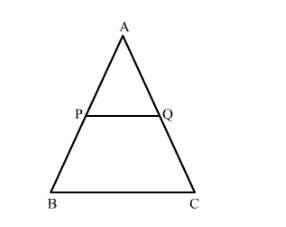# In a ∆ABC, P and Q are points on sides AB and AC respectively,

Question:

In a ∆ABC, P and Q are points on sides AB and AC respectively, such that PQ || BC. If AP = 2.4 cm, AQ = 2 cm, QC = 3 cm and BC = 6 cm, find the AB and PQ

Solution:It is given that $A P=2.4 \mathrm{~cm}, A Q=2 \mathrm{~cm}, Q C=3 \mathrm{~cm}$ and $B C=6 \mathrm{~cm}$.

We have to find $A B$ and $P Q$.

So $\frac{A P}{P B}=\frac{A Q}{Q C}$ (by Thales theorem)

Then $\frac{2.4}{P B}=\frac{2}{3}$

$\Rightarrow 2 P B=2.4 \times 3 \mathrm{~cm}$

$\Rightarrow P B=\frac{2.4 \times 3}{2} \mathrm{~cm}$

$=3.6 \mathrm{~cm}$

Now

$A B=A P+P B$

$=2.4+3.6 \mathrm{~cm}$

$=6 \mathrm{~cm}$

Since PQBC, AB is a transversal, then
∠APQ = ∠ABC  (corresponding angles)

Since PQBC, AC is a transversal, then
∠AQP = ∠ACB  (corresponding angles)

In ∆APQ and ∆ABC,

∠APQ = ∠ABC   (proved above)

∠AQP = ∠ACB   (proved above)

so, ∆ APQ ∼ ∆ ABC  (Angle Angle Similarity)

Since the corresponding sides of similar triangles are proportional, then

APAB = PQBC = AQAC

APAB = PQBC2.46 = PQ6so, PQ = 2.4 cm